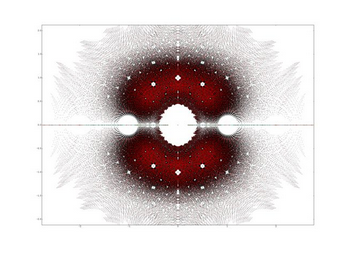# Compare the coefficients

Algebra Level 2Suppose the polynomials $P_1(x)$ and $P_2(x)$ are obtained by expanding and simplifying the following algebraic expressions

$P_1(1) = (1 + x^2 - x^5)^{2000} \\ P_2(x) = (1 - x^2 + x^5)^{2000}.$

Let $C_1$ and $C_2$ be the coefficient of $x^{800}$ in the polynomials $P_1(x)$ and $P_2(x)$ respectively. Which of the given option is true?

×